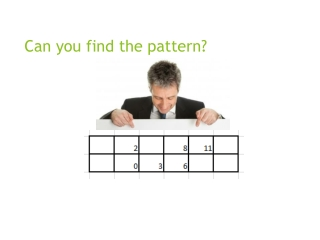DownloadDownload PresentationCan you find the pattern?

# Can you find the pattern?

Télécharger la présentation## Can you find the pattern?

- - - - - - - - - - - - - - - - - - - - - - - - - - - E N D - - - - - - - - - - - - - - - - - - - - - - - - - - -
##### Presentation Transcript

1. Can you find the pattern?

2. 3-2 Solving by Graphing Objective: to find the root/solution/zero of a linear equation by graphing.

3. Vocabulary • Root/ Zeros/ Solutions: the solutions of a equations; x-intercepts.

4. Linear Function

5. Example 1 B Method Solve by graphing. Using the x and y intercepts. B. Solve Where does the graph cross the x-axis?

6. Example 1 B The graph intersects the x-axis at –3. Answer: So, the solution is –3.

7. TOO Solve for the solution to the linear equation. A.x = –4 B.x = –9 C.x = 4 D.x = 9

8. A.x = 4; B.x = –4; C.x = –3; D.x = 3; Let’s try together Graph using the x and y intercepts.

9. What type of line would have no solution? So which line has no solution, y=3 or x=5? Explian your conclusion with your neighbor

10. Example 3 FUNDRAISING Kendra’s class is selling greeting cards to raise money for new soccer equipment. They paid \$115 for the cards, and they are selling each card for \$1.75. The function y = 1.75x – 115 represents their profit y for selling x greeting cards. Find the zero of this function. Describe what this value means in this context. The graph appears to intersect the x-axis at about 65. So…? That means what?

11. Example 3 Estimate by Graphing y = 1.75x – 115 Original equation 0 = 1.75x – 115 Replace y with 0. 115 = 1.75x Add 115 to each side. 65.71 ≈ x Divide each side by 1.75. Answer: The zero of this function is about 65.71. Since part of a greeting card cannot be sold, they must sell 66 greeting cards to make a profit.

12. Homework 3-2 Worksheet